Posted on

TL;DR: batch size 32 is probably going to be a good default candidate for many cases.

In this post, we will observe how different batch sizes change learning metrics when we train a model using Transfer Learning and the fast.ai library. We will try to find out which batch sizes are good and which are better to be avoided.

# Getting the dataset

Recently I was going through the awesome fast.ai deep learning course, and in one of the lectures we were building a classifier that can recognize cats and dogs. I wanted to build one too, and since I live in Hamburg I decided to go with some birds one can see there. Fortunately, there was a post by Luca Feuerriegel where I found the names of some of the species: European Robin, Marsh Tit, Eurasian Blackbird, Eurasian Nuthatch, Eurasian Jay, Eurasian Wren, Hawfinch, Bullfinch, Common Starling, Greylag Goose, Barnacle Goose, Meadow Pipit, Common Wood Pigeon, Mistle Thrush.

To collect the dataset, I used the bing image search to get images of every bird mentioned above. I'm not going to publish the collected images since I have doubts about violating copyright, however, you can download the source code of the notebook and run the experiment yourself.

After I collected the dataset, I did a few training iterations and cleaned the dataset using the `ImageClassifierCleaner` tool in the fast.ai library.

In the end, I ended up with 2017 pictures representing 14 different species of birds, which should be good enough to train the model fast and not overfit too quickly.

# Training the model

To obtain the results we're going to experiment with 3 ResNet architectures: ResNet50, ResNet34, and ResNet18. For each architecture, we will train the model 10 times with batch sizes of 128, 64, 32, 16, 8, and 4. We will also train the model for 10 epochs for each combination of the architecture and batch size.

We're also going to apply a few transformations and data augmentation steps to avoid overfitting: randomly cropping and resizing the images, and applying a standard set of batch augmentation (`aug_transforms()`):

``````birdsDB = DataBlock(
blocks=(ImageBlock, CategoryBlock),
get_items=get_image_files,
splitter=RandomSplitter(valid_pct=0.2, seed=1337),
get_y=parent_label,
item_tfms=RandomResizedCrop(224, min_scale=0.5),
batch_tfms=aug_transforms())
``````

The other parameters will be left to default. We will also use a `CSVLogger` callback to save the learning metrics to the CSV files which we will analyze later.

``````def train(arch, batch_size, index):
learn = cnn_learner(dls, architectures[arch], metrics=error_rate, cbs=[ShowGraphCallback, CSVLogger(fname=f'birds-{arch}-bs{batch_size}-{index}.csv')]).to_fp16()
learn.fine_tune(10)
``````

We will also train with half-precision to fit ResNet50 with 128 batch size into my GPU.

Finally this is our nested loop where we will try different parameters:

``````for arch in ['rn50', 'rn34', 'rn18']:
for bs in [128, 64, 32, 16, 8, 4]:
for index in range(10):
train(arch, bs, index)
torch.cuda.empty_cache()
gc.collect()
``````

It is worth noting that this loop took around 6 hours to finish, so be patient if you would like to experiment yourself :)

# Analyzing the results

After waiting for a few hours, we finally have all 180 CSV files ready for analysis. Yay!

Let's dig into them.

First we would need a function to parse the CSV file and convert it into a pandas dataframe:

``````def loadLog(bs: int, arch: str, idx: int) -> pd.DataFrame:
# change the time to seconds
# since no training epoch took more than 1 minute
# we will cheat and simply trim the minutes away
df['time'] = df.apply(lambda df: int(df['time'].split(':')), axis=1)
return df
``````

Then, because we have 10 dataframes per each combination of a batch size and an architecture, we will merge them together and calculate the average:

``````def loadMergedLog(bs: int, arch: str) -> pd.DataFrame:
dfs = map(lambda idx: loadLog(bs, arch, idx), range(10))
merged = pd.concat(dfs)
return merged.groupby(merged.index).mean()
``````

Finally, we need a function that will plot the results:

``````def plotResults(arch: str, y_axis: str):
fig, ax = plt.subplots()

fig.set_size_inches(20,15)

blockSizes = [128, 64, 32, 16, 8, 4]

for bs in blockSizes:
plt.plot(frame['epoch'], frame[y_axis])
ax.legend(blockSizes);

ax.legend(blockSizes);
plt.xlim(0,9)
plt.show()
``````

With all this in place, let's see how the batch size was affecting the training:

### ResNet50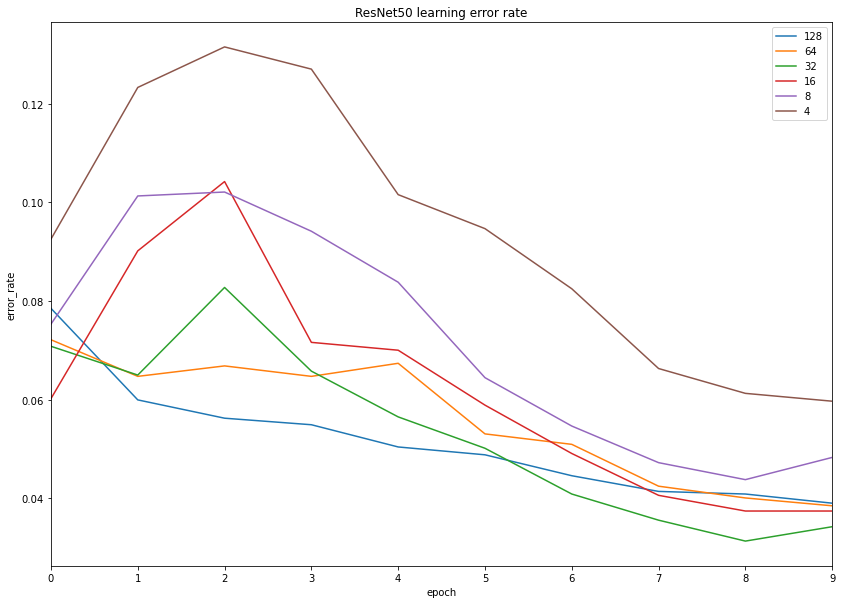Here we see that batch sizes 4 and 8 are not that good, and 32 gave us the lowest error after 10 epochs of training.

Now let's look at the training time: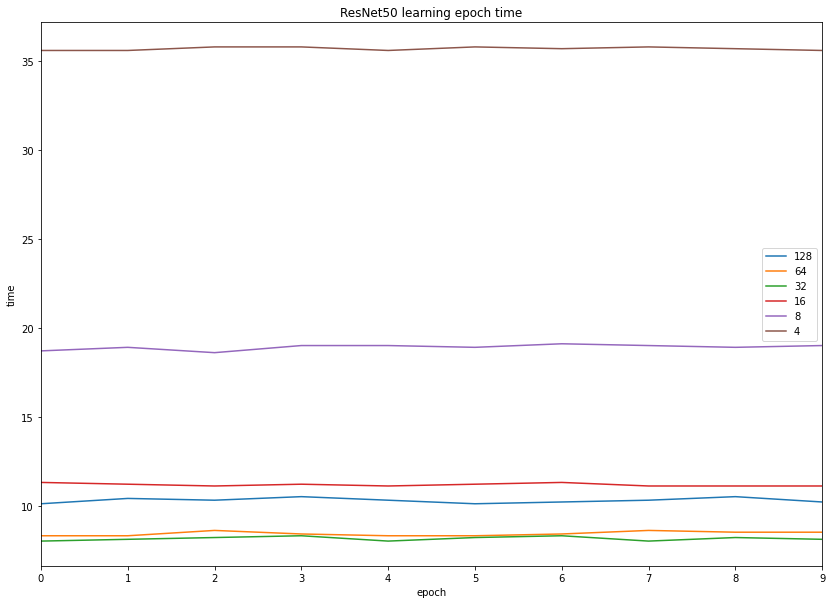Unsurprisingly, batch sizes 4 and 8 were slow due to copying overhead, while batch sizes of 32 and 64 were the fastest. Interestingly, a batch size of 128 was also slower than 32 and 64.

### ResNet34

With a reduced number of layers the model error rate seems to follow the same pattern as before: batch size 32 looking better than the others (however not that much) and batch size 4 again showed the lowest performance.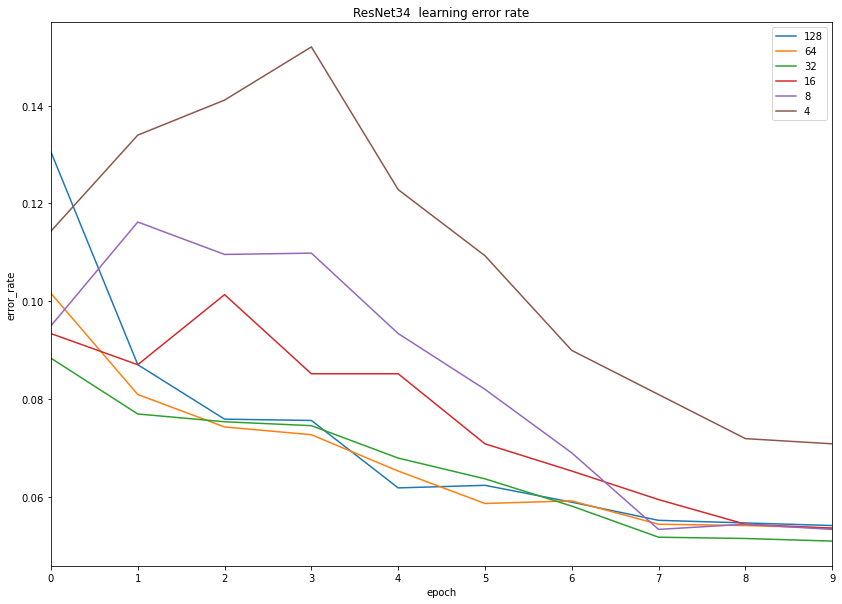Speaking of training time we see the same picture: batch sizes of 32 and 64 being the fastest, and 4 being the slowest.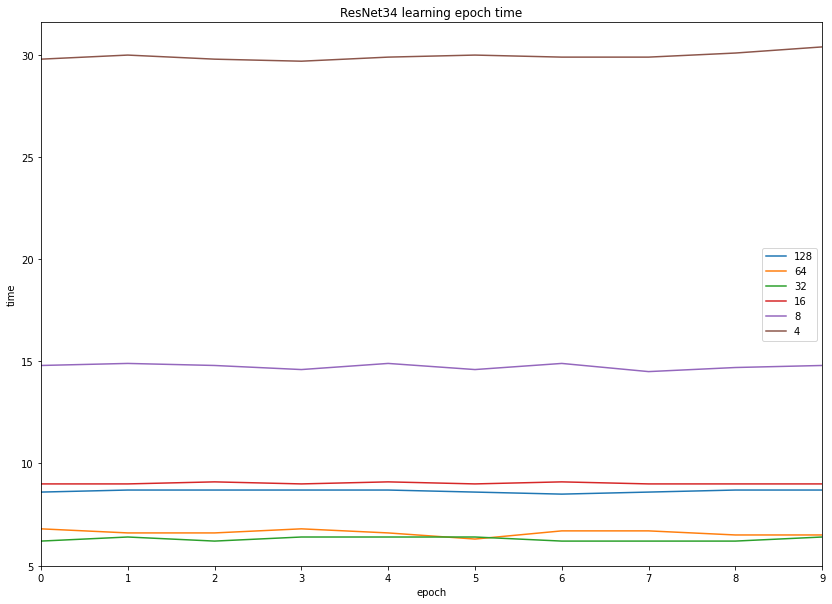Initially, the learning performance doubles when we double the batch size (bs16 is twice as fast as bs8, and bs8 is twice as fast as bs4), and stabilizes around 32 and 62 images per batch.

### ResNet18

When we train the model using an even smaller ResNet architecture, our previous results are confirmed again: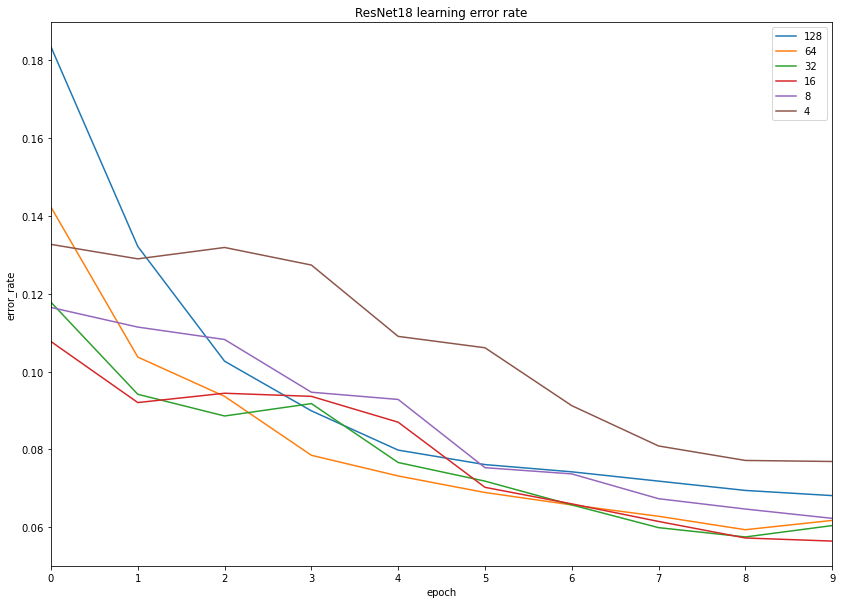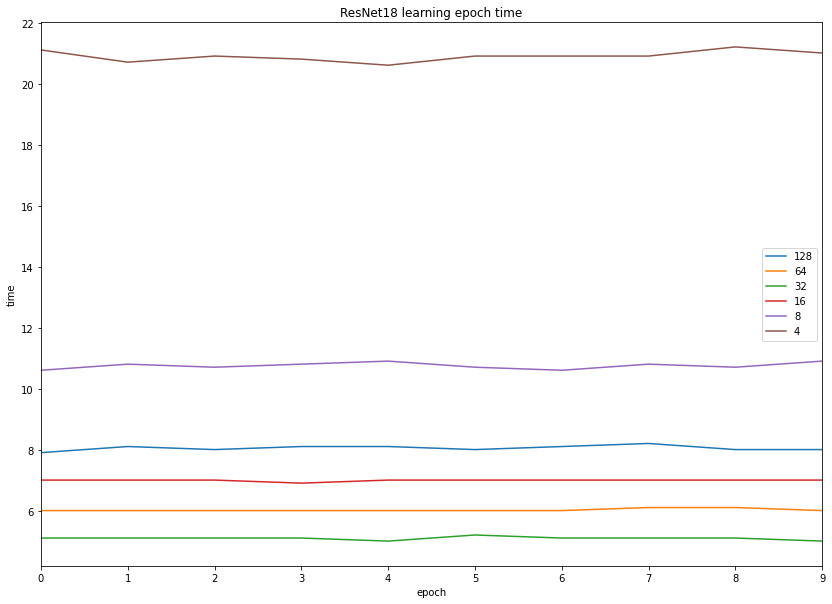Learning was the fastest with batch size 32, and the performance of all three 16, 32, and 64 batch sizes are very similar.

# Results

We trained the classifier on the natural images resized to 224 pixels, and discovered that batch size 32 was often surpassing other candidates in terms of learning speed and error rate.

This means that it is probably going to be a good default candidate when we try to analyze natural images and want to iterate quickly, for example when wanting to clean up the dataset.

Batch sizes of 8 and less are probably better be avoided if your images are small due to high overhead on data transfer.

Training with a batch size of 128 was slower and a bit less accurate, so it might not be the ideal candidate to start with.